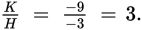# SAT Math Multiple Choice Question 328: Answer and Explanation

### Test Information

Question: 328

13.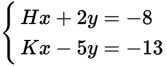If the solution to the system of equations shown above is (2, –1), what is the value of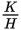?

• A. –3
• B. –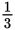• C.• D. 3

Explanation:

D

Difficulty: Hard

Category: Heart of Algebra / Systems of Linear Equations

Strategic Advice: Typically, solving a system of equations means finding the values of x and y that satisfy both equations simultaneously. In this question, you already know that. The question may look complicated, but don't let it intimidate you. Because the solution to the system satisfies both equations, you can substitute 2 and -1, for x and y respectively, and then solve for H and K. Before selecting your answer, check that you found what the question was asking for (the value of).

Getting to the Answer: Substitute the values of x and y into each equation and solve for H and K. Then, divide K by H.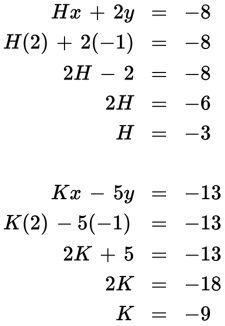So,